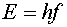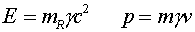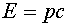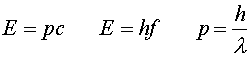Photons as light quanta A photon is a quanta of light. Our picture of light, to this point, has been that of a wave. Wave-like characteristics are responsible for diffraction and refraction. However, light is absorbed and emitted one photon at a time. The energy in a photon is related to the frequency of the light wave through Planck's constant.Photons are massless particles. The idea of massless particles may seem a bit strange. To understand them better, we consider the expressions for energy and momentum of particles of mass m.Massless particles can be thought of as the limit of massive particles with m approaching zero. Even though the mass may be infinitesimally small, the factor g may approach infinity as the velocity approaches c. Thus for massless particles, the above expressions are meaningless, but the ratio of the two expressions is valid as the factors g and m cancel.We have made use of the fact that the velocity v equals c for massless particles. By adding the usual relation of wavelength and frequency, c = lf, one gets three useful expressions relating energy, momentum, frequency and wavelength.Thus light of shorter wavelengths, interacts as photons with higher energy than does light with longer wavelengths. We will later discuss the fact that the latter two expressions remain true for massive particles, although the energy in the central expression includes the rest energy, (1/2)mc2.